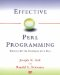# Item 6: Understand conversions between strings and numbers.

### Item 6: Understand conversions between strings and numbers .

Perl's scalar variables can contain either string or numeric data. They can also contain both at the same time, usually as the result of converting string data to a number, or vice versa.

Perl automatically converts values from numeric to string representation, or vice versa, as required. For example, if a string appears next to a numeric operator like + , Perl converts the string value to a number before proceeding with the arithmetic. Or, if a number is the object of a pattern match, Perl first converts the number to a string. Places where strings are expected are referred to as string contexts , and places where numbers are expected are referred to as numeric contexts . These are nice terms to know, but we won't use them very often in this book, since it rarely makes any real difference.

The function used for converting numbers to strings is the C standard library's sprintf() , with a format of "%.15g" or something similar.  You can change this format by changing the special variable \$# , but the use of \$# is deprecated. If you need to use a particular format, use Perl's sprintf :

 Well, sort of. Perl prefers to use gconvert() . This is one of the reasons you shouldn't mess with \$# any more.

 ` \$n = sprintf "%10.4e", 3.1415927; ` ` "3.1416e+00" `

The function used for converting strings to numbers is the C standard library's atof() . Any leading white space is ignored. Conversion uses whatever leading part of the string appears number-like, and the rest is ignored. Anything that doesn't look like a number is converted to zero. For example:

 ` \$n = 0 + "123"; \$n = 0 + "123abc"; \$n = 0 + "\n123"; \$n = 0 + "a123"; ` 123 Also 123 trailing stuff ignored. Also 123 leading whitespace. no number at beginning.

The conversion process does not recognize octal or hexadecimal. Use the oct operator to convert octal or hexadecimal strings:

 ` \$n = 0 + "0x123"; \$n = 0 + oct("0x123"); ` looks like number . 291 oct converts octal and hex strings to decimal. ` print "mode (octal): "; chmod , \$file; ` Prompt for file mode. WRONGstring from STDIN converted to decimal, not octal. ` print "mode (octal): "; chmod oct(), \$file; ` Prompt for file mode. RIGHTmode string converted to octal.

When a number is automatically converted to a string, or vice versa, both representations remain . They will persist until the value of the variable is changed.

Usually, it does not matter whether a variable contains a string or a numeric value, but there are a few occasions when it does. For example, the bitwise numeric operators act on the whole numeric value if applied to a number, but characterwise if applied to a string:

 ` \$a = 123; \$b = 234; \$c = \$a & \$b; ` number 106 ` \$a = "\$a"; \$b = "\$b"; \$d = \$a & \$b; ` string "020"

Finally, the error variable \$! is an example of a variable with a " magic" property. It returns the value of the system variable errno when it is used in a numeric context, but it returns the string from the perror() function (or some equivalent for your system) in a string context:

 ` open FH, ""; ` Invalid filename; should produce an error. ` print "\$!\n"; ` "No such file or directory" ` print 0 + \$!, "\n"; ` "2" (or whatever)Effective Perl Programming: Writing Better Programs with Perl
ISBN: 0201419750
EAN: 2147483647
Year: 1996
Pages: 116

Similar book on Amazon